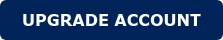Planar Magnetics Design: Two-Dimensional Winding Losses Calculation

Planar magnetics are increasingly used in switch-mode power supplies (SMPSs) due to their unique advantages, so accurate losses models are needed to avoid using finite element methods (FEM) in the magnetic elements design procedure, due to the fact that a lot of computing time is required.

The objective of this App Note is to compare two winding losses calculation models: the classical Dowell one-dimensional (1-D) model and a two-dimensional (2-D) model, that takes the edge effect into consideration.Conductor Losses per meter Calculation when a Sinusoidal Waveform is Applied

1-D conductor losses model: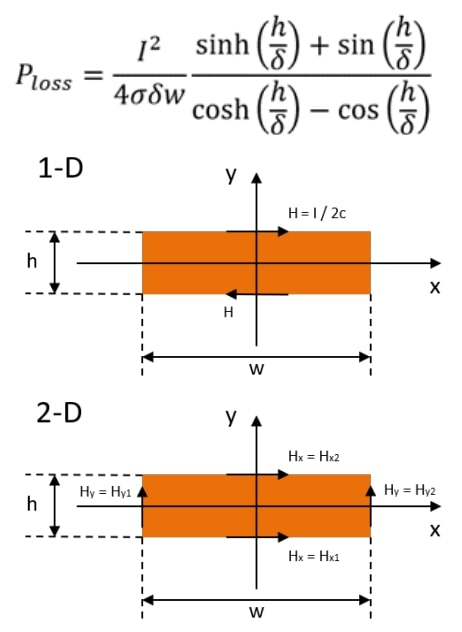2-D conductor losses model:

Unlike the 1-D model, it considers the conductor thickness, so the two longitudinal components of the magnetic field strength affect the winding losses value and a more accurate result is obtained.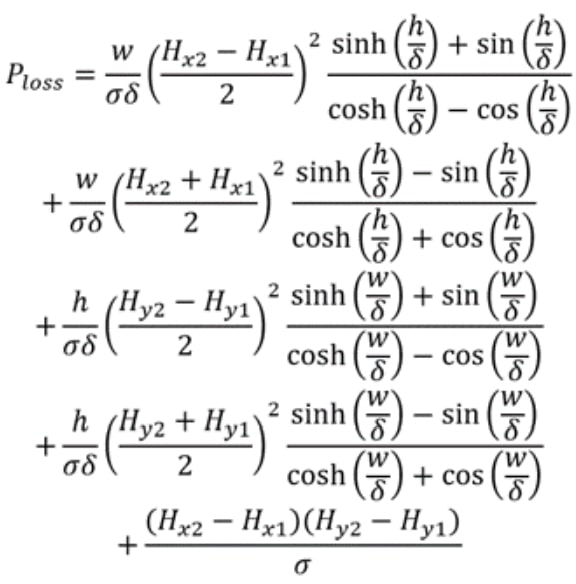Where:

• I is the sinusoidal current amplitude.
• w is the conductor width.
• h is the conductor thickness.
• δ is the skin depth shown in the image below: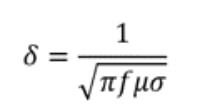• σ is the conductor conductivity.
• H is the magnetic field strength.
• f is the operating frequency.

Winding Losses Calculation using both 1-D and 2-D Models in a 1 Layer and 3 Layers Inductor: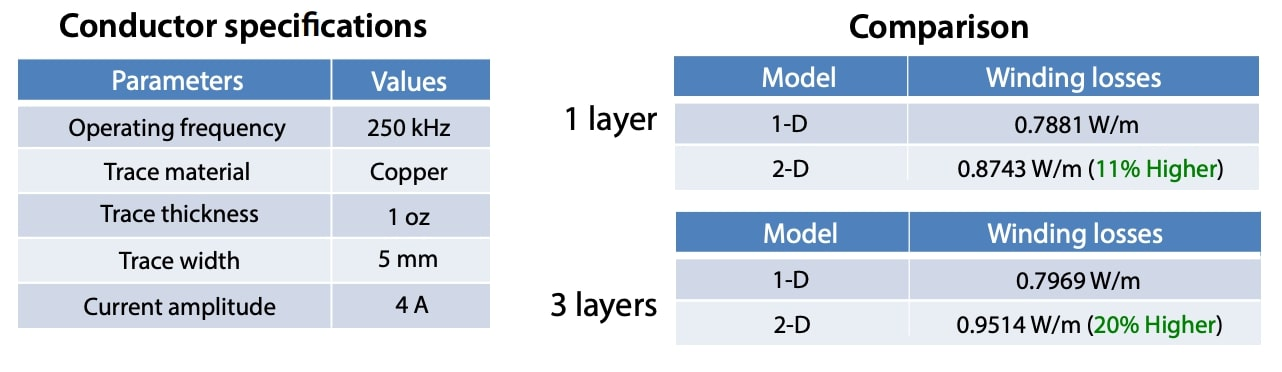It can be seen how the conductor thickness influence in the winding losses is not negligible and the losses difference between both models increase with the number of layers.

Conclusions

The winding losses calculation is an important step in the magnetic design procedure, and a high difference between theoretical and real results can be shown if a low accurate model is used. Frenetic, thanks to its AI technology, is able to use real measurements during the magnetic elements design, for having more accurate results without using FEM.

You may also like